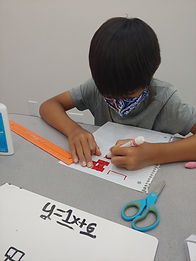## Ms. Mari

### Target 1​

###### Lesson Type:

Continuation

Algebra

:

Variable

Write a linear equation in slope-intercept form.

###### 1:

Use the graph of a linear equation to identify slope and y-intercept.

###### 2:

Identify the equation y = mx + b as defining a linear function which graphs a straight line.

###### 3:

Recognize m as the slope.

###### 4:

Recognize b as the y-intercept.

8th

###### Vocabulary:

SLOPE, Y-INTERCEPT, SLOPE-INTERCEPT FORM

Activities:

- Given examples of linear equations, students created a table of values for each example by identifying three ordered pairs (x, y) that are on the line, one of which was the y-intercept (when x = 0).

- Students then used the table of values to graph the linear equation.

- Lastly, students identified the slope and y-intercept of the line, then wrote an equation in slope-intercept form (y = mx + b).### Home Exploration

###### Guiding Questions:## Absent Students:

Jacob

### Target 2

:

###### 1:

Generate growing patterns.

8th

###### Vocabulary:

SLOPE-INTERCEPT FORM, GROWING PATTERN, SLOPE, Y-INTERCEPT

Activities:

Given a linear equation in slope-intercept form, students created a growing pattern to match the equation and interpreted the meaning of the slope and y-intercept.### Home Exploration

###### Guiding Questions:### Target 3

:

###### Vocabulary:

Activities:### Home Exploration